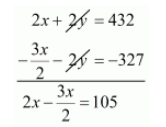# A railway half ticket costs half the full fare and the reservation charge is the same on half ticket as on full ticket.`
Question:

A railway half ticket costs half the full fare and the reservation charge is the same on half ticket as on full ticket. One reserved first class ticket from Mumbai to Ahmedabad costs Rs 216 and one full and one half reserved first class tickets cost Rs 327. What is the basic first class full fare and what is the reservation charge?

Solution:

Let take first class full of fare is Rs $x$ and reservation charge is Rs $y$ per ticket

Then half of the ticket as on full ticket $=\frac{x}{2}$

According to the given condition we have

$x+y=216 \cdots(i)$

$x+y+\left(\frac{x}{2}\right)+y=327$

$x+\frac{x}{2}+y+y=327$

$\frac{3 x}{2}+2 y=327 \cdots(i i)$

Multiplying equationby 2 we have

$2 x+2 y=432 \cdots(\mathrm{iii})$

Subtracting $(i i)$ from $(i i i)$ we get$\frac{4 x-3 x}{2}=105$

$\frac{1 x}{2}=105$

$x=105 \times 2$

$x=210$

Putting $x=210$ in equation $(i)$ we get

$x+y=216$

$210+y=216$

$y=216-210$

$y=6$

Hence, the basic first class full fare is 210

The reservation charge is Rs 6 .